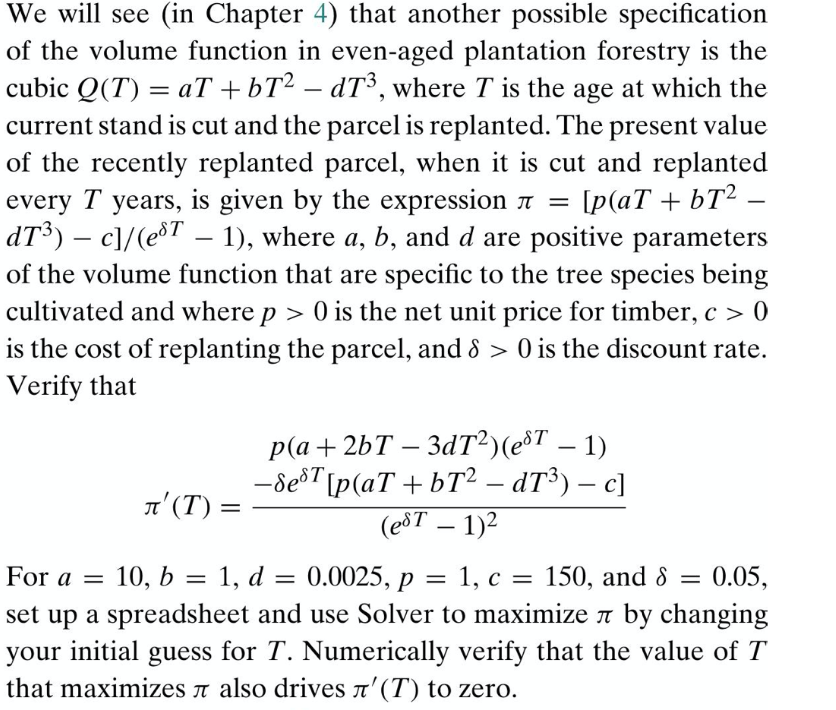### Create an Account

Already have account?

### Forgot Your Password ?

Home / Questions / We will see (in Chapter 4) that another possible specification of the volume function in e...

# We will see (in Chapter 4) that another possible specification of the volume function in even-aged plantation forestry is the cubic Q(T) = aT +bT? – dT3, where T is the age at which the current stand

We will see (in Chapter 4) that another possible specification of the volume function in even-aged plantation forestry is the cubic Q(T) = aT +bT? – dT3, where T is the age at which the current stand is cut and the parcel is replanted. The present value of the recently replanted parcel, when it is cut and replanted every T years, is given by the expression r = [p(aT + b12 - dT3) – c]/(e&T – 1), where a, b, and d are positive parameters of the volume function that are specific to the tree species being cultivated and where p > 0 is the net unit price for timber, c > 0 is the cost of replanting the parcel, and 8 > 0 is the discount rate. Verify that p(a + 2bT – 3dT?)(eST – 1) -8e8T[p(aT +bT2 – dT3) – c] #'(T) = (eðT – 1)2 10, b = 1, d = 0.0025, p = 1, c = 150, and 8 0.05, set up a spreadsheet and use Solver to maximize a by changing your initial guess for T. Numerically verify that the value of T that maximizes n also drives a' (T) to zero. For a = =Apr 16 2021 View more View Less

#### Answer (Solved)Subscribe To Get Solution